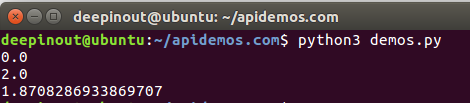# Python Math pow() 函数

## Python Math pow() 函数

Python Math pow() 方法返回 xy（x的y次方） 的值。

## Python Math pow() 语法

``````import math

math.pow( x, y )``````

``pow(x, y[, z])``

• x - 数值表达式。
• y - 数值表达式。
• z - 数值表达式。

## Python Math pow() 实例

``````#!/usr/bin/python3
import math

print ("math.pow(100, 2) : ", math.pow(100, 2))
# 使用内置，查看输出结果区别
print ("pow(100, 2) : ", pow(100, 2))
print ("math.pow(100, -2) : ", math.pow(100, -2))
print ("math.pow(2, 4) : ", math.pow(2, 4))
print ("math.pow(3, 0) : ", math.pow(3, 0))``````• 微信订阅
• 回顶